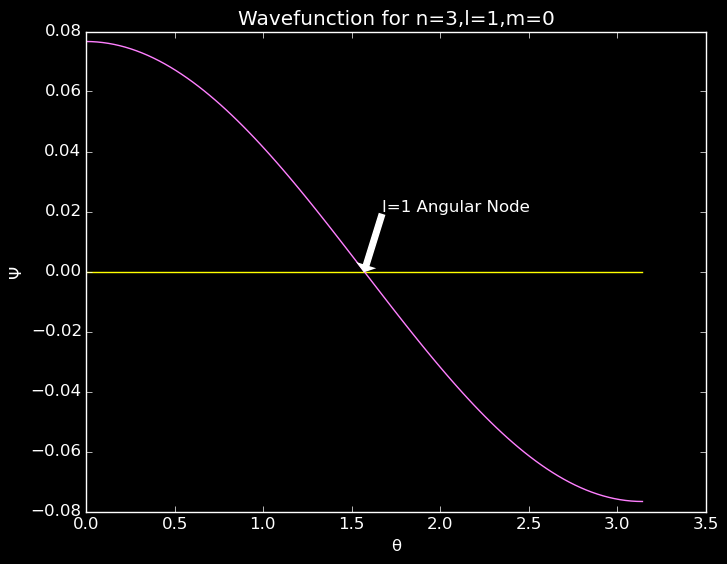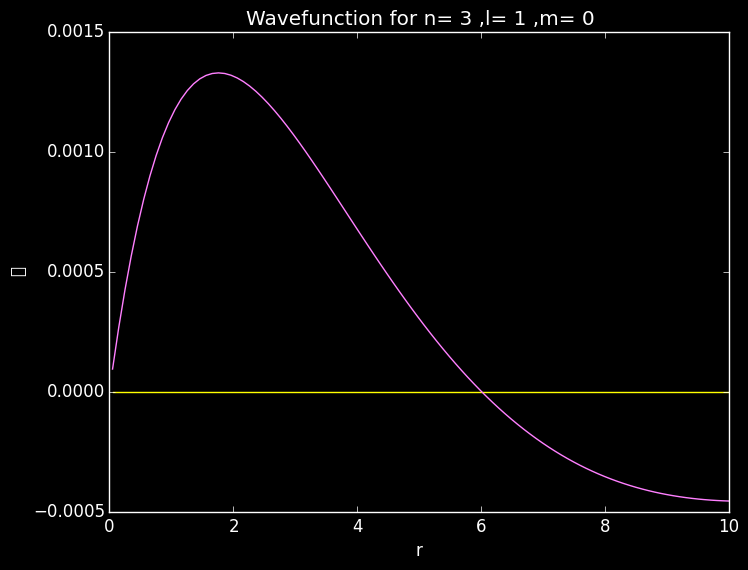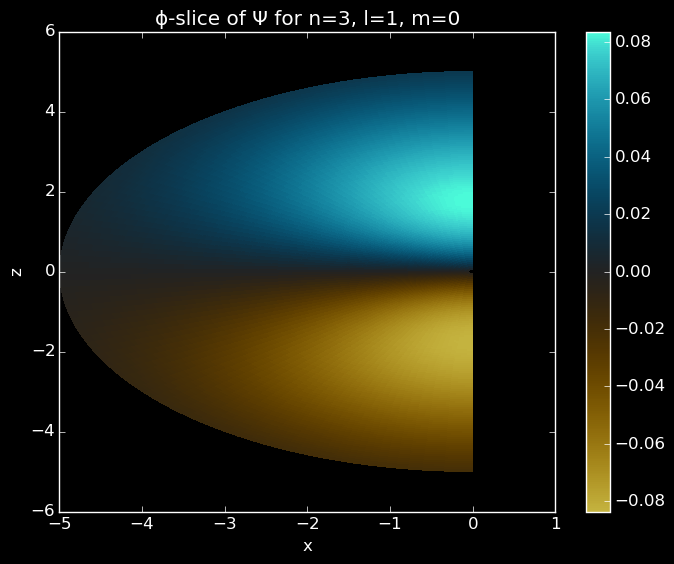Prerequiresites: Quantum Mechanics course

Electrons around a nucleus. Do they look like little well behaved planets orbiting a sun?

NOPE!

We get spread out blobs in special little patterns called orbitals. Here, we will look at their shapes and properties a bit. Today we will look at graphs in 1D and 2D, but the next post, Atomic Orbitals Pt. 2, uses a fancy, but slightly unstable plotting package, GLVisualize to generate some 3D plots.

The Hamiltonian for our problem is:

\begin{equation} {\cal H}\Psi(\mathbf{x}) =\left[ -\frac{\hbar}{2 m} \nabla^2 - \frac{Z e^2}{4 \pi \epsilon_0 r}\right]\Psi(\mathbf{x}) = E \Psi(\mathbf{x}) \end{equation} with \begin{equation} \nabla^2= \frac{1}{r^2}\frac{\partial}{\partial r} \left( r^2 \frac{\partial}{\partial r} \right)+ \frac{1}{r^2 \sin \theta} \frac{\partial}{\partial \theta} \left( \sin \theta \frac{\partial}{\partial \theta} \right)+ \frac{1}{r^2 \sin^2 \theta} \frac{\partial^2}{\partial \phi^2} \end{equation}

To solve this problem, we begin by guessing a solution with separated radial and angular variables, \begin{equation} \Psi(\mathbf{x}) = R(r) \Theta ( \theta,\phi) \end{equation}

\begin{equation} \frac{E r^2 R(r)}{2r R^{\prime}(r) + r^2 R^{\prime \prime}(r)}= \frac{\left( \frac{1}{\sin \theta} \frac{\partial}{\partial \theta} \left( \sin \theta \frac{\partial \Theta(\theta,\phi)}{\partial \theta} \right)+ \frac{1}{\sin^2 \theta} \frac{\partial^2 \Theta(\theta,\phi)}{\partial \phi^2}\right) }{\Theta( \theta, \phi)} =C \end{equation}

Instead of going into the precise mechanisms of solving those two separate equations here, trust for now that they follow standard special functions, the associated Legendre polynomial and the generalized Laguerre polynomial. Try a standard quantum mechanics textbook for more information about this.

\begin{equation} Y^m_l(θ,ϕ) = (-1)^m e^{i m \phi} P^m_l (\cos(θ)) \end{equation} where $P^m_l (\cos (\theta))$ is the associated Legendre polynomial.

\begin{equation} R^{n,l} ( \rho ) = \rho ^l e^{- \rho /2} L^{2 l+1}_{n-l-1} ( \rho ) \end{equation} where $L^{2 l+1}_{n-l-1}(\rho)$ is the generalized Laguerre polynomial.

\begin{equation} \rho=\frac{2r}{n a_0} \end{equation}

\begin{equation} N=\sqrt{\left(\frac{2}{n}\right)^3 \frac{(n-l-1)}{2n(n+l)!}} \end{equation}

Cell to Evaluate

What’s below is a bunch of definitions that makes our calculations easier later on. Here I utilize the GNU scientific library, GSL imported above, to calculate the special functions.

Programming Tip!

Even though it's not necessary, specifying the type of inputs to a function through m::Int helps prevent improper inputs and allows the compiler to perform additional optimizations. Julia also implements abstract types, so we don't have to specify the exact type of Int. Real allows a numerical, non-complex type.

Type Greek characters in Jupyter notebooks via LaTeX syntax, e.g. \alpha+tab

The function Orbital throws DomainError() when l or m do not obey their bounds. Julia supports a wide variety of easy to use error messages.

Parameters

Grid parameters: You might need to change rmax to be able to view higher $n$ orbitals.

Remember that \begin{equation} 0<n \;\;\;\;\; \;\;\;\; 0 \leq l < n \;\;\;\;\; \;\;\;\; -l \leq m \leq l \;\;\;\;\; \;\;\;\; n,l,m \in {\cal Z} \end{equation}

Though I could create a wrapped up function with Orbital(n,l,m) and evaluate that at each point, the below evaluation takes advantage of the separability of the solution with respect to spherical dimensions. The special functions, especially for higher modes, take time to calculate, and the fewer calls to GSL, the faster the code will run. Therefore, this implementation copies over radial and angular responses.A slice along the θ plane showing an angular node for the 2p orbital.A slice along the radial plane showing a radial node in the 3p orbital.Slice of a 3p orbital in the x and z plane.Slice of a 3dz2 orbital in the x and z plane.

Don’t forget to check out Atomic Orbitals Pt. 2!